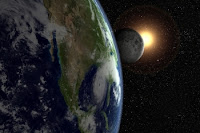## Saturday, 18 August 2012

### Order of magnitude

An order of magnitude is a geometric scale increasing or decreasing by the ratio of 10. It is applied to various measurements such as distance, money, or even just numerical value.The distance to the moon is 400,000 km, to the sun is 150,000,000 km. The ratio between these is 375, thus the sun is more than 2 orders of magnitude (more than 100×) further away from the earth than the moon is. The diameter of the earth is 12,740 km and diameter is 40,000 km. The moon is an order of magnitude (~10×) further from the earth than the distance of circumnavigating the earth.

My issue with orders of magnitude is while useful, a ratio of 10 seems very large and completely arbitrary. It relates to our use of base-10 (decimal), which probably derives from our pentadactyly.

A more natural scale for orders of magnitude would be binary. This is the lowest practical ratio. An order of magnitude larger would be twice the size, 2 orders of magnitude 4 times the size.

Of course a constant multiplier, be it 2 or 10, represents a logarithmic function. Order of magnitude being logarithmic may be better modelled on the natural logarithm. Using base e (~2.71828), while difficult numerically, would mean that the scale of magnitude is ~2.7.

A thousand-fold difference is 3 orders of magnitude (decimal). It would be ~10 orders of magnitude using binary, or ~7 orders or magnitude with base-e.